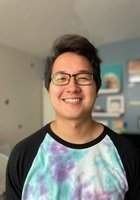1 of 7

# Connect with hundreds of tutors like Casey

Expert tutoring for students at all levels

150,000+ clients. 4.9 / 5.0 Rating of Tutoring Sessions

Featured by
Tutors from# Casey

Certified Tutor

I am a graduate from Arizona State University with a BS in Mathematics and MA in Applied Mathematics. I have over 5 years experience as a tutor and graduate teaching assistant. I've taught and/or assisted courses including Calculus, MATLAB, Differential Equations, and more. I was the lead tutor of mathematics at our engineering tutoring center, where we applied inquiry-based learning in a one-on-one tutoring environment similar to Varsity Tutors.

#### Casey’s Qualifications

Education & Certification

Undergraduate Degree: Arizona State University - Bachelor of Science, Mathematics

Graduate Degree: Arizona State University - Master of Arts, Applied Mathematics

Test Scores

GRE Quantitative: 167

Hobbies

My mathematical interests include numerical methods/analysis, linear algebra, real analysis, partial/ordinary differential equations, and calculus. I also enjoy programming in Matlab/Octave, Python, and good ol' Fortran. My hobbies apart from math include playing Super Smash Bros. Melee, exercising, and cooking.

Tutoring Subjects

Algebra

Algebra 2

AP Calculus AB

AP Calculus BC

Calculus

Calculus 2

Calculus 3

Calculus and Vectors

Differential Equations

Discrete Math

Linear Algebra

Math

MATLAB

Multivariable Calculus

Numerical Analysis

Productivity

Real Analysis

Technology and Coding

Trigonometry